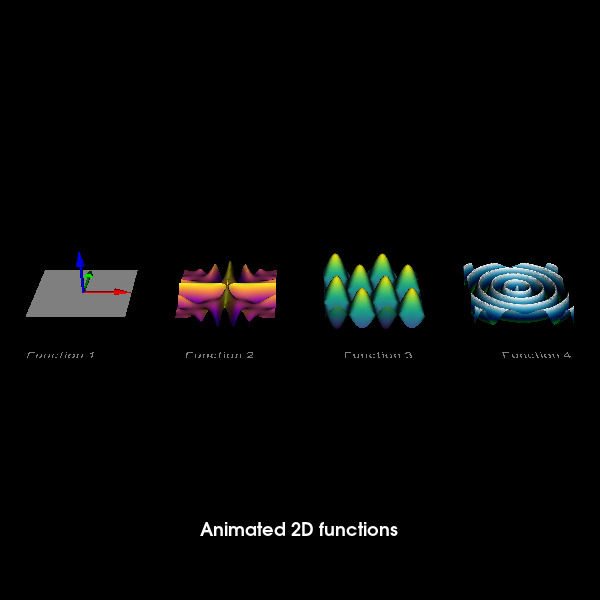# Animated 2D functions#

This is a simple demonstration of how one can animate 2D functions using FURY.

Importing necessary modules

```import itertools

import numpy as np

from fury import actor, colormap, ui, utils, window
```

The following function is used to create and update the coordinates of the points which are being used to plot the surface. It’s also used to create and update the colormap being used to color the surface. Kindly note that only the z coordinate is being modified with time as only the z coordinate is a function of time.

```def update_surface(x, y, equation, cmap_name='viridis'):

# z is the function F i.e. F(x, y, t)
z = eval(equation)
xyz = np.vstack([x, y, z]).T

# creating the colormap
v = np.copy(z)
m_v = np.max(np.abs(v), axis=0)
v /= m_v if m_v else 1
colors = colormap.create_colormap(v, name=cmap_name)

return xyz, colors
```

Variables and their usage: :time - float: initial value of the time variable i.e. value of the time variable at

the beginning of the program; (default = 0)

dt: float

amount by which `time` variable is incremented for every iteration of timer_callback function (default = 0.1)

lower_xbound: float

lower bound of the x values in which the function is plotted (default = -1)

upper_xbound: float

Upper bound of the x values in which the function is plotted (default = 1)

lower_ybound: float

lower bound of the y values in which the function is plotted (default = -1)

upper_ybound: float

Upper bound of the y values in which the function is plotted (default = 1)

npoints: int

For high quality rendering, keep the number high but kindly note that higher values for npoints slows down the animation (default = 128)

```time = 0
dt = 0.1
lower_xbound = -1
upper_xbound = 1
lower_ybound = -1
upper_ybound = 1
npoints = 128
```

creating the x, y points which will be used to fit the equation to get elevation and generate the surface

```x = np.linspace(lower_xbound, upper_xbound, npoints)
y = np.linspace(lower_ybound, upper_ybound, npoints)
x, y = np.meshgrid(x, y)
x = x.reshape(-1)
y = y.reshape(-1)
```

Function used to create surface obtained from 2D equation.

```def create_surface(x, y, equation, colormap_name):
xyz, colors = update_surface(x, y, equation=equation, cmap_name=colormap_name)
surf = actor.surface(xyz, colors=colors)
surf.equation = equation
surf.cmap_name = colormap_name
surf.vertices = utils.vertices_from_actor(surf)
surf.no_vertices_per_point = len(surf.vertices) / npoints**2
surf.initial_vertices = surf.vertices.copy() - np.repeat(
xyz, surf.no_vertices_per_point, axis=0
)
return surf
```

Equations to be plotted

```eq1 = 'np.abs(np.sin(x*2*np.pi*np.cos(time/2)))**1*np.cos(time/2)*\
np.abs(np.cos(y*2*np.pi*np.sin(time/2)))**1*np.sin(time/2)*1.2'
eq2 = '((x**2 - y**2)/(x**2 + y**2))**(2)*np.cos(6*np.pi*x*y-1.8*time)*0.24'
eq3 = '(np.sin(np.pi*2*x-np.sin(1.8*time))*np.cos(np.pi*2*y+np.cos(1.8*time)))\
*0.48'
eq4 = 'np.cos(24*np.sqrt(x**2 + y**2) - 2*time)*0.18'
equations = [eq1, eq2, eq3, eq4]
```

List of colormaps to be used for the various functions.

```cmap_names = ['hot', 'plasma', 'viridis', 'ocean']
```

Creating a list of surfaces.

```surfaces = []
for i in range(4):
surfaces.append(
create_surface(x, y, equation=equations[i], colormap_name=cmap_names[i])
)
```

Creating a scene object and configuring the camera’s position

```scene = window.Scene()
scene.set_camera(
position=(4.45, -21, 12), focal_point=(4.45, 0.0, 0.0), view_up=(0.0, 0.0, 1.0)
)
showm = window.ShowManager(scene, size=(600, 600))
```

Creating a grid to interact with surfaces individually.

```# To store the function names
text = []
for i in range(4):
t_actor = actor.vector_text(
'Function ' + str(i + 1), pos=(0, 0, 0), scale=(0.17, 0.2, 0.2)
)
text.append(t_actor)

grid_ui = ui.GridUI(
actors=surfaces,
captions=text,
caption_offset=(-0.7, -2.5, 0),
dim=(1, 4),
aspect_ratio=1,
rotation_axis=(0, 1, 0),
)

# Adding an axes actor to the first surface.
```

Initializing text box to print the title of the animation

```tb = ui.TextBlock2D(bold=True, position=(200, 60))
tb.message = 'Animated 2D functions'
```

Initializing showm and counter

```counter = itertools.count()
```

end is used to decide when to end the animation

```end = 200
```

The 2D functions are updated and rendered here.

```def timer_callback(_obj, _event):
global xyz, time
time += dt
cnt = next(counter)

# updating the colors and vertices of the triangles used to form the
# surfaces
for surf in surfaces:
xyz, colors = update_surface(
x, y, equation=surf.equation, cmap_name=surf.cmap_name
)
utils.update_surface_actor_colors(surf, colors)
surf.vertices[:] = surf.initial_vertices + np.repeat(
xyz, surf.no_vertices_per_point, axis=0
)
utils.update_actor(surf)

showm.render()
# to end the animation
if cnt == end:
showm.exit()
```

Run every 30 milliseconds

```showm.add_timer_callback(True, 30, timer_callback)

interactive = False
if interactive:
showm.start()

window.record(showm.scene, size=(600, 600), out_path='viz_animated_surfaces.png')
```Total running time of the script: ( 0 minutes 0.533 seconds)

Gallery generated by Sphinx-Gallery